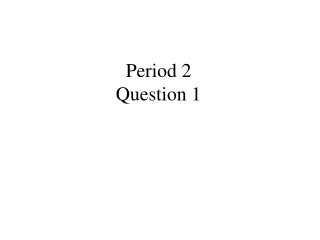DownloadDownload PresentationPeriod 2 Question 1

# Period 2 Question 1

Download Presentation## Period 2 Question 1

- - - - - - - - - - - - - - - - - - - - - - - - - - - E N D - - - - - - - - - - - - - - - - - - - - - - - - - - -
##### Presentation Transcript

1. Period 2Question 1

2. The kinetic energy of an object is increased 8 times as a result of: • Increasing the mass 4 times • Increasing the speed 4 times • Increasing both the mass and the speed 2 times • Increasing the speed 8 times • Applying a force which is 8 times greater

3. Period 2Question 2

4. When an object is dropped from a tower, what is the effect of the air resistance? • Increases the objects kinetic energy • Increases the objects potential energy • Does positive work on the object • Decreases the objects mechanical energy • None of the above is correct

5. Period 3Question

6. The kinetic energy of an object is increased by a factor of 27 by: • Decreasing the mass 3 times and increasing the speed 3 times • Increasing the mass 3 times and increasing the speed 3 times • Increasing the speed 27 times and keeping the mass constant • Decreasing the mass 27 times and increasing the speed 9 times • None of the above is correct

7. Period 3 Question 2

8. Two blocks (heavy and light) are released from the top of a building. The light falls straight down and the heavy slides down a ramp. If all friction is ignored, which one is moving faster when they reach the bottom? • heavy • light • both move with the same speed at the bottom • depends on the slide shape • insufficient information given